Next: Electric Quadrupole Transitions Up: Time-Dependent Perturbation Theory Previous: Forbidden Transitions

# Magnetic Dipole Transitions

According to Equation (8.195), the quantity that mediates spontaneous magnetic dipole transitions between different atomic states is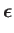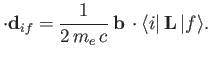(8.194)

However, it turns out that the previous expression is incomplete because, in writing the Hamiltonian (8.128), we neglected to take into account the interaction of the magnetic component of the electromagnetic wave with the electron's magnetic moment. This interaction gives rise to an additional term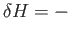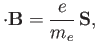(8.195)

where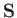is the electron spin. (See Section 5.5.) Now,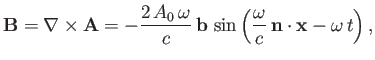(8.196)

where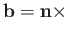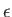, and use has been made of Equation (8.130). It follows that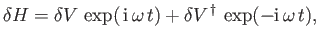(8.197)

where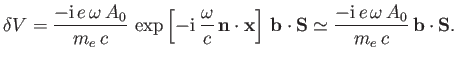(8.198)

Hence, according to Equation (8.137), there is an addition contribution to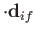of the form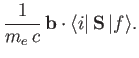(8.199)

(Here, we have again assumed that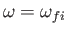.) In other words, the complete quantity that mediates magnetic dipole transitions between different atomic states is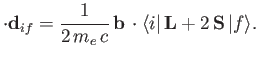(8.200)

It follows, by analogy with Equation (8.165), that the spontaneous emission rate associated with a magnetic dipole transition is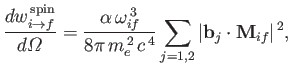(8.201)

where(8.202)

is termed the magnetic dipole matrix element, and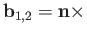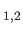. Here,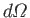is the solid angle associated with the direction of the emitted photon's normalized wavevector,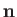. Moreover,are the photon's two independent electric polarization vectors. In fact, it is easily demonstrated that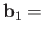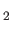, and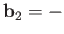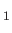. Hence, Equation (8.204) can also be written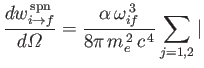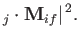(8.203)

It follows that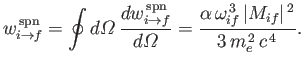(8.204)

(See Exercise 8.)

According to the analysis of Chapters 4 and 5,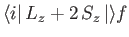is only non-zero for a hydrogen-like atom if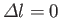,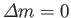, and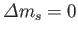. Here,is the azimuthal quantum number,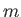the magnetic quantum number, and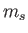the spin quantum number. Likewise,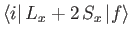and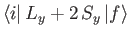are only non-zero if,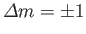, and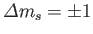. Hence, the selection rules for magnetic dipole transitions are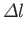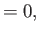(8.205)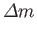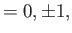(8.206)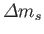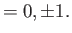(8.207)

Consider the hydrogen atom. Let us calculate the magnetic dipole matrix element,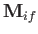, for the case where the initial state is characterized by the quantum numbers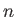,,, and, and the final state is characterized by the quantum numbers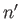,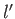,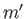, and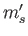. Because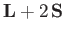has no dependance on the radial variable, the radial component of this matrix element is simply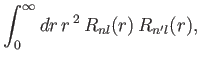(8.208)

where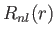is a standard hydrogen radial wavefunction. Here, we have made use of the fact that the matrix element is zero unless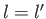. However, according to Exercise 17, the previous integral is zero unless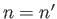: that is, unless the initial and final states have the same energy. Hence, we conclude that magnetic dipole transitions between different energy levels (i.e., atomic states corresponding to different values of the principle quantum number,) of a hydrogen atom are forbidden.

A more general form of the selection rules (8.208)-(8.210) is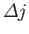(8.209)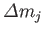(8.210)

where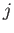and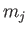are the standard quantum numbers associated with the total angular momentum of the system. Note, however, that a magnetic dipole transition between two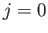states is forbidden. These new rules suggest that it is possible to have a magnetic dipole transition between hydrogen atom states whose energies are split by spin-orbit effects: for instance, between a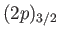and a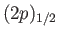state. (See Section 7.7.) Let us estimate the typical spontaneous emission rate for such a transition. We expect the matrix element, defined in Equation (8.205), to be of order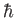. We also expect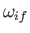to be of order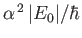, where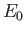is the hydrogen ground-state energy. (See Exercise 14.) It thus follows from Equation (8.207) that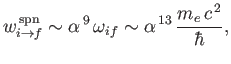(8.211)

which much smaller than a typical electric dipole transition rate. [See Equation (8.180) and Exercise 16.]

The most celebrated example of a magnetic dipole transition in physics is that which mediates the spontaneous decay of the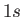triplet state of a hydrogen atom to the corresponding singlet state. (See Section 7.9.) This transition, which takes place at a very slow rate (i.e.,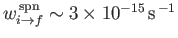--see Exercise 18), produces the well-known hydrogen 21 cm line.Next: Electric Quadrupole Transitions Up: Time-Dependent Perturbation Theory Previous: Forbidden Transitions
Richard Fitzpatrick 2016-01-22